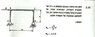# Statics helps - should be easy question

• Femme_physics
In summary, you solved for the vertical componency at A...there is a horizontal component also.Sum of all forces on X: F - Ra = 0Ra = FRa^2 = F^2 + 0.5F^2 Ra = Root of F + 0.25F

#### Femme_physics

Gold Member
I really appreciate you guys helping me in all those problems - tiny-tim, nvn! I appreciate the fact there's online support to help me get ready as I'm about to go into a study group with other students which I'll probably be the teacher of (they haven't solved most of what I solved partly thanks to you). I want to get everything straight.

## Homework Statement

http://img256.imageshack.us/img256/4048/52987150.jpg [Broken]

Calculate the forces at the supports A and D of this hard frame depicted in the diagram. On the frame acts at point B horizontal force F. No need to consider the frame's weight.

The answers are written in the scanjob at the lower right section.

## The Attempt at a Solution

Okay, I get Rd: Tell me if it's correct

Sum of all moments on A = 0 = -F x a + D x 2a = 0
Rd = Fa/2a
Rd = 0.5 F

Okay, got it.

Sum of all moments on D = -F x a + Ray x 2a = 0
Fa = Ray x 2a
Ray = Fa/2a
Ray = 0.5 F

Okay...getting the same result in both...what's wrong with this picture?

#### Attachments

•zz.jpg
24.6 KB · Views: 395
Last edited by a moderator:
Dory said:
I really appreciate you guys helping me in all those problems - tiny-tim, nvn! I appreciate the fact there's online support to help me get ready as I'm about to go into a study group with other students which I'll probably be the teacher of (they haven't solved most of what I solved partly thanks to you). I want to get everything straight.

## Homework Statement

http://img256.imageshack.us/img256/4048/52987150.jpg [Broken]

Calculate the forces at the supports A and D of this hard frame depicted in the diagram. On the frame acts at point B horizontal force F. No need to consider the frame's weight.

The answers are written in the scanjob at the lower right section.

## The Attempt at a Solution

Okay, I get Rd: Tell me if it's correct

Sum of all moments on A = 0 = -F x a + D x 2a = 0
Rd = Fa/2a
Rd = 0.5 F

Okay, got it.

Sum of all moments on D = -F x a + Ray x 2a = 0
Fa = Ray x 2a
Ray = Fa/2a
Ray = 0.5 F

Okay...getting the same result in both...what's wrong with this picture?
You solved for the vertical componeny at A...there is a horizontal component also.

Last edited by a moderator:
Sum of all forces on X: F - Ra = 0
Ra = F

Ra^2 = F^2 + 0.5F^2
Ra = Root of F + 0.25F

I know I'm missing something obvious...darn it. More help?

Dory said:
Sum of all forces on X: F - Ra = 0
Ra = F

Ra^2 = F^2 + 0.5F^2
using Pythagorus, that should be
Ra^2 = F^2 + (0.5F)^2
Ra^2 = F^2 + 0.25F^2
Ra^2 = 1.25F^2
Ra^2 = (5/4)(F^2)
Ra = sq. rt[(5/4)(F^2)]
Ra = [(sq rt 5)/2]F
I know I'm missing something obvious...darn it. More help?
It's your algebra, so I tried to work it out for you step by step...your Physics behind the solution is correct..watch your maths. You could get the same answer by noting that the resultant is the hypotenuse of a 1:2:rt5 right triangle.
Note that often rather than combine the components into a resultant magnitude (and direction!), it is often best and acceptable to leave the answer in it x and y vector components...also note direction of component force reactions (left or right , up or down)?

Ra^2 = F^2 + 0.25F^2

Wait, after you raised 0.5F to the power of 2, and you got 0.25F, the ^2 should be erased - why is it still there? You've already raised it by the exponent at this point... See, this is the main part that confuses me...

I can't believe I blundered the algebra! First time I see unknown in pythagoras though.. I'll rework that again and see what I'm missing, thanks for the reply PhantomJ. :)

Dory said:
Wait, after you raised 0.5F to the power of 2, and you got 0.25F, the ^2 should be erased - why is it still there? You've already raised it by the exponent at this point... See, this is the main part that confuses me...
F is a letter variable with a numerical solution...you should treat it like a number. If
y = (ab)2, then
y = a2b2. Similarly, if

y = (0.5F)2, then
y = (0.5)2F2, or
y = 0.25F^2

Q.E.D. (quod erat demonstrandum)